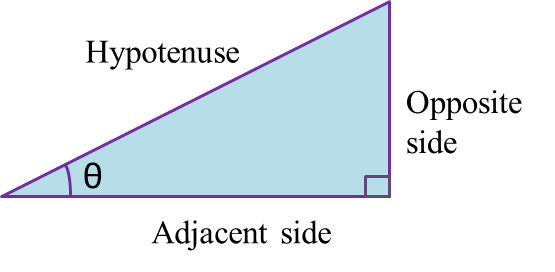# 5.1.1 Trigonometric Ratios

5.1 Trigonometric Ratios

5.1.1 Trigonometrical Ratios of an Acute Angle

1.2.   Hypotenuse is the longest side of the right-angled triangle which is opposite the right angle.

3.   Adjacent side is the side, other than the hypotenuse, which has direct contact with the given angle, θ.

4.   Opposite side is the side which is opposite the given angle, θ.
5.   In a right-angled triangle,

6. When the size of an angle θ increases from 0o to 90o,
· sin θ increases.
· cos θ increases.
· tan θ increases.

7.   The values of sin θ, cos θ and tan θ remain the same even though the size of the triangle has changed.
Example:Find the sine, cosine and tangent of the give angle, θ, in triangle ABC.

Solution:
$\begin{array}{l}\mathrm{sin}\theta =\frac{BC}{AB}=\frac{8}{17}\\ \mathrm{cos}\theta =\frac{AC}{AB}=\frac{15}{17}\\ tan\theta =\frac{BC}{AC}=\frac{8}{15}\end{array}$